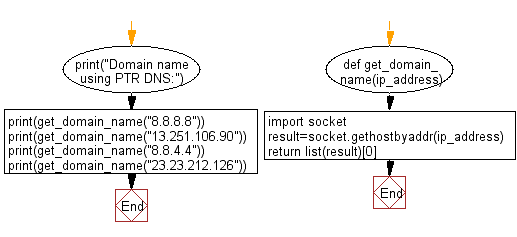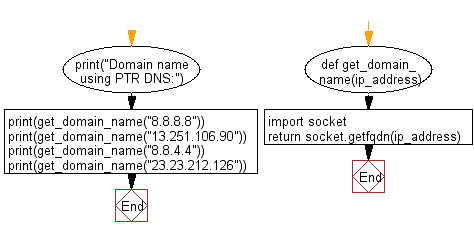﻿ Python: Get the domain name using PTR DNS records from a given IP address - w3resource# Python: Get the domain name using PTR DNS records from a given IP address

## Python Basic - 1: Exercise-141 with Solution

Write a Python program to get the domain name using PTR DNS records from a given IP address.

What is a DNS PTR record?

The Domain Name System, or DNS, correlates domain names with IP addresses. A DNS pointer record (PTR for short) provides the domain name associated with an IP address. A DNS PTR record is exactly the opposite of the 'A' record, which provides the IP address associated with a domain name.

DNS PTR records are used in reverse DNS lookups. When a user attempts to reach a domain name in their browser, a DNS lookup occurs, matching the domain name to the IP address. A reverse DNS lookup is the opposite of this process: it is a query that starts with the IP address and looks up the domain name. Source: cloudflare.com

Sample Solution-1:

Python Code:

``````def get_domain_name(ip_address):
import socket
return list(result)
print("Domain name using PTR DNS:")
print(get_domain_name("8.8.8.8"))
print(get_domain_name("13.251.106.90"))
print(get_domain_name("8.8.4.4"))
print(get_domain_name("23.23.212.126"))
``````

Sample Output:

```Domain name using PTR DNS:
ec2-13-251-106-90.ap-southeast-1.compute.amazonaws.com
ec2-23-23-212-126.compute-1.amazonaws.com
```

Flowchart:## Visualize Python code execution:

The following tool visualize what the computer is doing step-by-step as it executes the said program:

Sample Solution-2:

Python Code:

``````def get_domain_name(ip_address):
import socket
print("Domain name using PTR DNS:")
print(get_domain_name("8.8.8.8"))
print(get_domain_name("13.251.106.90"))
print(get_domain_name("8.8.4.4"))
print(get_domain_name("23.23.212.126"))
``````

Sample Output:

```Domain name using PTR DNS:
ec2-13-251-106-90.ap-southeast-1.compute.amazonaws.com
ec2-23-23-212-126.compute-1.amazonaws.com
```

Flowchart:## Visualize Python code execution:

The following tool visualize what the computer is doing step-by-step as it executes the said program:

Python Code Editor:

Have another way to solve this solution? Contribute your code (and comments) through Disqus.

What is the difficulty level of this exercise?

Test your Programming skills with w3resource's quiz.

﻿

## Python: Tips of the Day

What is the difference between Python's list methods append and extend?

append: Appends object at the end.

```x = [1, 2, 3]
x.append([4, 5])
print (x)
```

Output:

```[1, 2, 3, [4, 5]]
```

extend: Extends list by appending elements from the iterable.

```x = [1, 2, 3]
x.extend([4, 5])
print (x)
```

Output:

```[1, 2, 3, 4, 5]
```

Ref: https://bit.ly/2AZ6ZFq